# Counters in Digital Logic

• Difficulty Level : Medium
• Last Updated : 28 Jun, 2021

According to Wikipedia, in digital logic and computing, a Counter is a device which stores (and sometimes displays) the number of times a particular event or process has occurred, often in relationship to a clock signal. Counters are used in digital electronics for counting purpose, they can count specific event happening in the circuit. For example, in UP counter a counter increases count for every rising edge of clock. Not only counting, a counter can follow the certain sequence based on our design like any random sequence 0,1,3,2… .They can also  be designed with the help of flip flops.

Counter Classification

Attention reader! Don’t stop learning now. Get hold of all the important CS Theory concepts for SDE interviews with the CS Theory Course at a student-friendly price and become industry ready.

Counters are broadly divided into two categories

1. Asynchronous counter
2. Synchronous counter

1. Asynchronous Counter

In asynchronous counter we don’t use universal clock, only first flip flop is driven by main clock and the clock input of rest of the following flip flop is driven by output of previous flip flops. We can understand it by following diagram-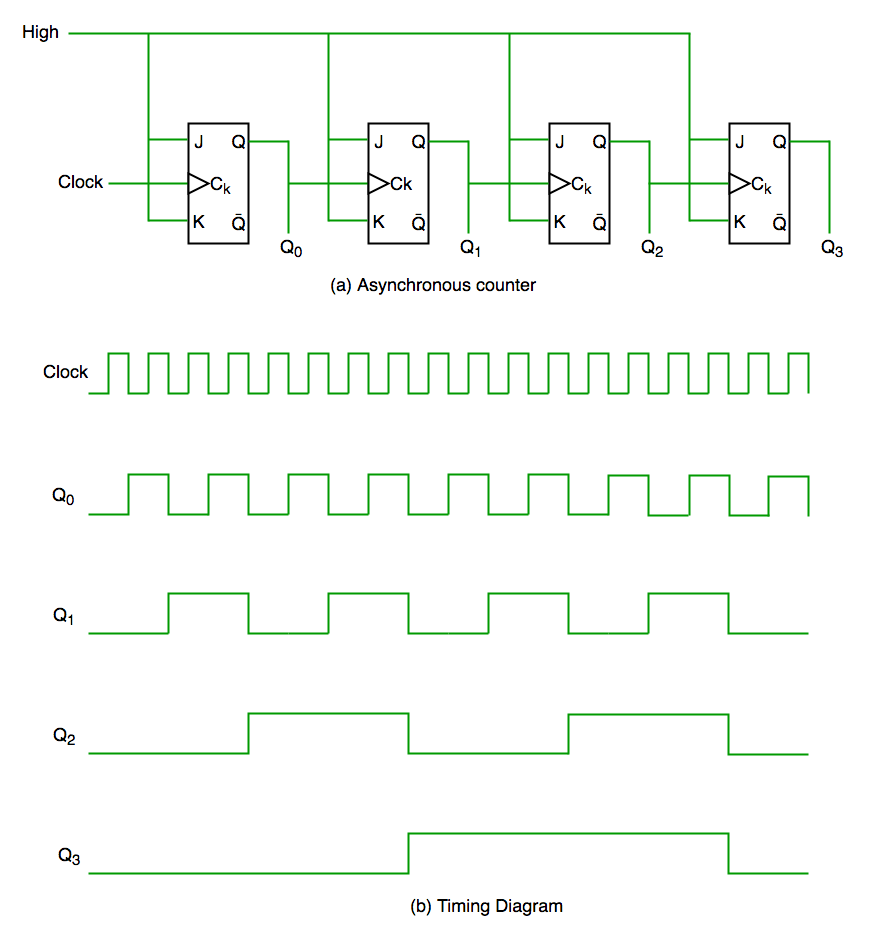It is evident from timing diagram that Q0 is changing as soon as the rising edge of clock pulse is encountered, Q1 is changing when rising edge of Q0 is encountered(because Q0 is like clock pulse for second flip flop) and so on. In this way ripples are generated through Q0,Q1,Q2,Q3 hence it is also called RIPPLE counter.

2. Synchronous Counter

Unlike the asynchronous counter, synchronous counter has one global clock which drives each flip flop so output changes in parallel. The one advantage of synchronous counter over asynchronous counter is, it can operate on higher frequency than asynchronous counter as it does not have cumulative delay because of same clock is given to each flip flop.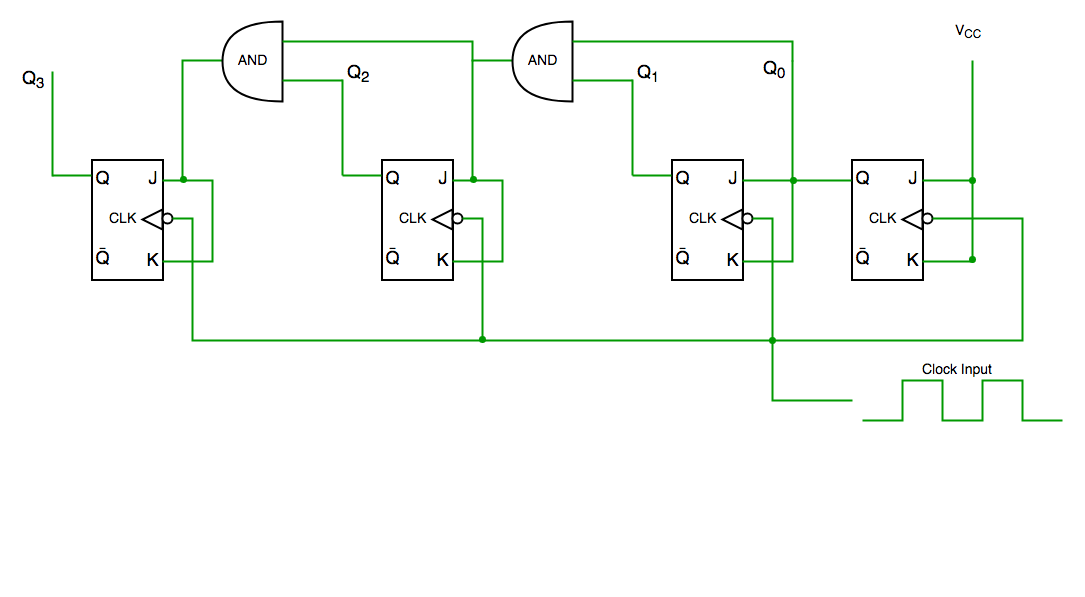Synchronous  counter circuitTiming diagram synchronous counter

From circuit diagram we see that Q0 bit gives response to each falling edge of clock while Q1 is dependent on Q0, Q2 is dependent on Q1 and Q0 , Q3 is dependent on Q2,Q1 and Q0.

A decade counter counts ten different states and then reset to its initial states. A simple decade counter will count from 0 to 9 but we can also make the decade counters which can go through any ten states between 0 to 15(for 4 bit counter).

 Clock pulse Q3 Q2 Q1 Q0 0 0 0 0 0 1 0 0 0 1 2 0 0 1 0 3 0 0 1 1 4 0 1 0 0 5 0 1 0 1 6 0 1 1 0 7 0 1 1 1 8 1 0 0 0 9 1 0 0 1 10 0 0 0 0

Truth table for simple decade counter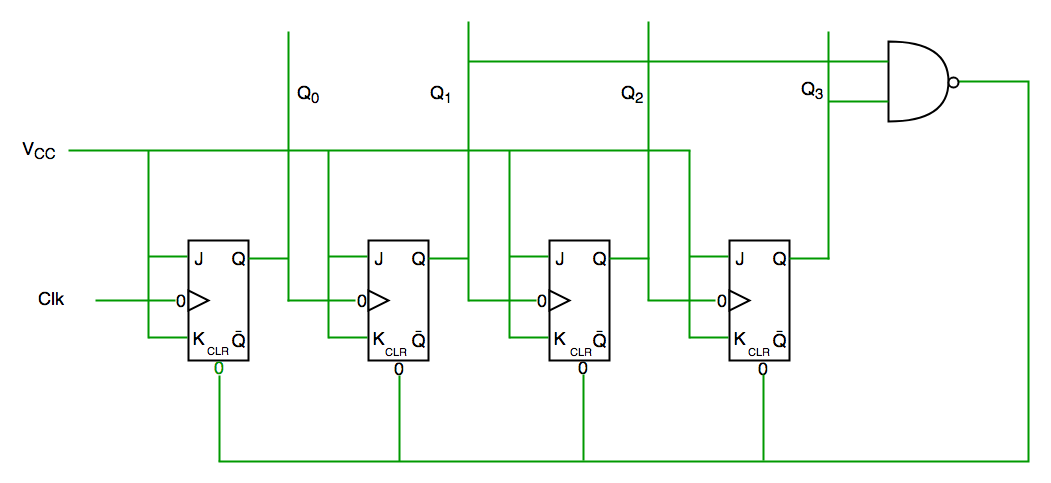We see from circuit diagram that we have used nand gate for Q3 and Q1 and feeding this to clear input line because binary representation of 10 is—

1010

And we see Q3 and Q1 are 1 here, if we give NAND of these two bits to clear input then counter will be clear at 10 and again start from beginning.

Important point: Number of flip flops used in counter are always greater than equal to (log2 n)  where n=number of states in counter.

Some previous years gate questions on Counters

Q1. Consider the partial implementation of a 2-bitt counter using T flip-flops following the sequence 0-2-3-1-0, as shown below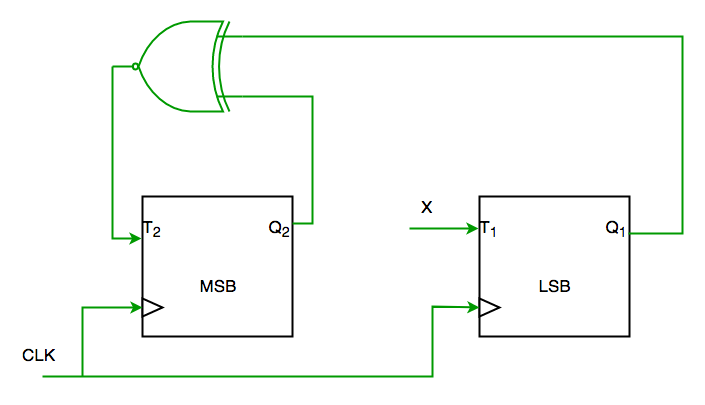To complete the circuit, the input X should be

(A) Q2?
(B) Q2 + Q1
(C) (Q1 ? Q2)’
(D) Q1 ? Q2                                                                                         (GATE-CS-2004)

Solution:

From circuit we see

T1=XQ1’+X’Q1—-(1)

AND

T2=(Q2 ? Q1)’—-(2)

AND DESIRED OUTPUT IS 00->10->11->01->00

SO X SHOULD BE Q1Q2’+Q1’Q2 SATISFYING 1 AND 2.

SO ANS IS (D) PART.

Q2. The control signal functions of a 4-bit binary counter are given below (where X is “don’t care”)
The counter is connected as follows: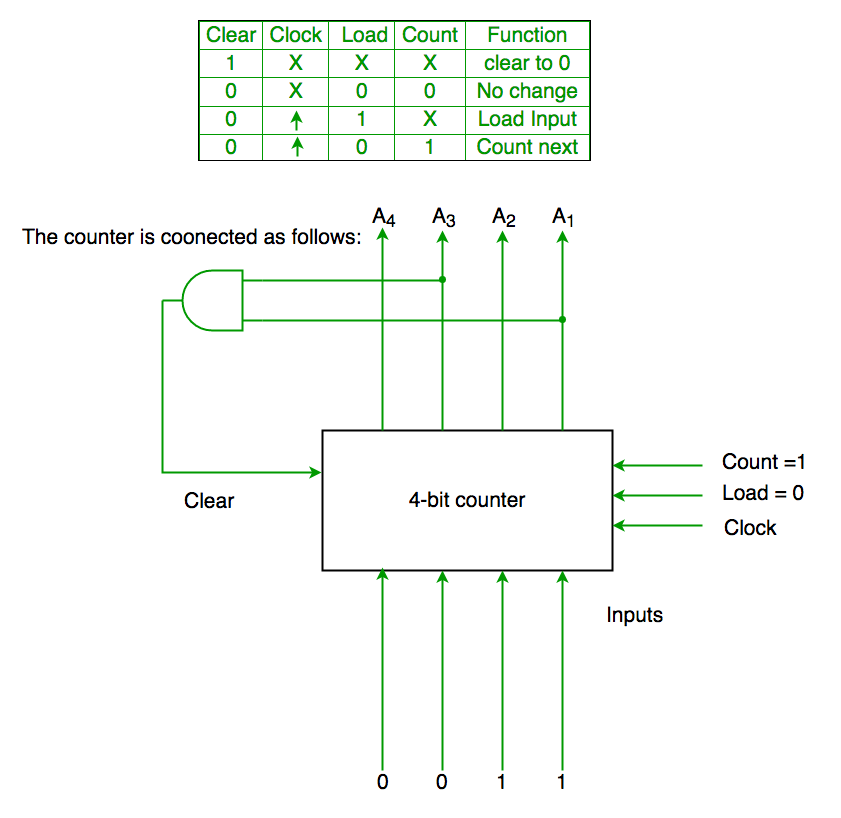Assume that the counter and gate delays are negligible. If the counter starts at 0, then it cycles through the following sequence:

(A) 0,3,4

(B) 0,3,4,5

(C) 0,1,2,3,4

(D) 0,1,2,3,4,5                                                                                                            (GATE-CS-2007)

Solution:

Initially A1 A2 A3 A4 =0000

Clr=A1 and A3

So when A1 and A3 both are 1 it again goes to 0000

Hence 0000(init.) -> 0001(A1 and A3=0)->0010 (A1 and A3=0) -> 0011(A1 and A3=0) -> 0100 (A1 and A3=1)[ clear condition satisfied] ->0000(init.) so it goes through 0->1->2->3->4

Ans is (C) part.

Quiz on Digital Logic